# Golden ratio facts for kids

Kids Encyclopedia Facts

With one number a and another smaller number b, the ratio of the two numbers is found by dividing them. Their ratio is a/b. Another ratio is found by adding the two numbers together and dividing this by the larger number a. The new ratio is (a+b)/a. If these two ratios are equal to the same number, then that number is called the golden ratio. The Greek letter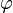$\varphi$ (phi) is usually used as the name for the golden ratio.

For example, if b = 1 and a/b =$\varphi$, then a =$\varphi$. The second ratio (a+b)/a is then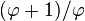$(\varphi+1)/\varphi$. Because these two ratios are equal, this is true: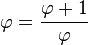$\varphi = \frac{\varphi+1}{\varphi}$

One way to write this number is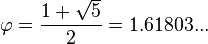$\varphi = \frac{1 + \sqrt{5}}{2} = 1.61803...$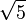$\sqrt{5}$ is like any number which, when multiplied by itself, makes 5 (or which number is multiplied):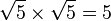$\sqrt5\times\sqrt5=5$.

The golden ratio is an irrational number. If a person tries to write it, it will never stop and never make a pattern, but it will start this way: 1.6180339887... An important thing about this number is that a person can subtract 1 from it or divide 1 by it. Either way, the number will still keep going and never stop.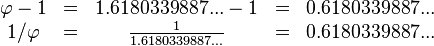$\begin{array}{ccccc} \varphi-1 &=& 1.6180339887...-1 &=& 0.6180339887...\\ 1/\varphi &=& \frac{1}{1.6180339887...} &=& 0.6180339887... \end{array}$

## Golden rectangle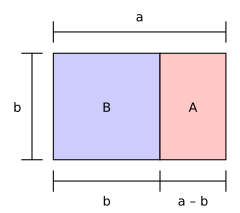The large rectangle BA is a golden rectangle; that is, the proportion b:a is 1:$\varphi$. For any such rectangle, and only for rectangles of that specific proportion, if we remove square B, what is left, A, is another golden rectangle; that is, with the same proportions as the original rectangle.

If the length of a rectangle divided by its width is equal to the golden ratio, then the rectangle is a "golden rectangle". If a square is cut off from one end of a golden rectangle, then the other end is a new golden rectangle. In the picture, the big rectangle (blue and pink together) is a golden rectangle because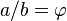$a/b=\varphi$. The blue part (B) is a square, and the pink part by itself (A) is another golden rectangle because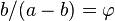$b/(a-b)=\varphi$. The big rectangle and the pink rectangle have the same form, but the pink rectangle is smaller and is turned.

## Fibonacci numbers

The Fibonacci numbers are a list of numbers. A person can find the next number in the list by adding the last two numbers together. If a person divides a number in the list by the number that came before it, this ratio comes closer and closer to the golden ratio.

Fibonacci number divided by the one before ratio
1
1 1/1 = 1.0000
2 2/1 = 2.0000
3 3/2 = 1.5000
5 5/3 = 1.6667
8 8/5 = 1.6000
13 13/8 = 1.6250
21 21/13 = 1.6154...
34 34/21 = 1.6190...
55 55/34 = 1.6177...
89 89/55 = 1.6182...
... ... ...$\varphi$ = 1.6180...

## Golden ratio in nature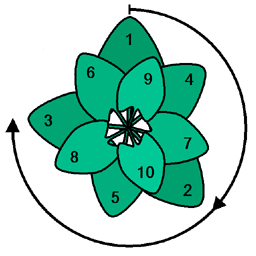Using the golden angle will optimally use the light of the sun. This is a view from the top.

In nature, the golden ratio is often used for the arrangement of leaves or flowers. These use the golden angle of approximately 137.5 degrees. Leaves or flowers arranged in that angle best use sunlight.

## Images for kidsGolden ratio Facts for Kids. Kiddle Encyclopedia.